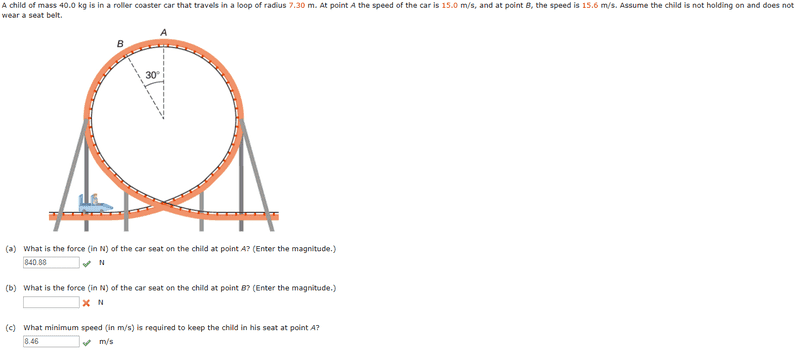# Roller Coaster Force Question

• Austin Gibson
In summary, the question asks for the force of the car seat on the child at point B, given the child's mass, the car's speed at points A and B, and the radius of the loop. The student attempted to solve it by using the equation Fc = [(mv^2)/r * cos30] - mg, but incorrectly applied cos30 to the gravitational force instead of the centripetal force. The correct equation is Fc = [(mv^2)/r] * cos30 - mg.f

## Homework Statement

:[/B]
A child of mass 40.0 kg is in a roller coaster car that travels in a loop of radius 7.30 m. At point A the speed of the car is 15.0 m/s, and at point B, the speed is 15.6 m/s. Assume the child is not holding on and does not wear a seat belt.

What is the force (in N) of the car seat on the child at point B? (Enter the magnitude.)Fc = [(mv^2)/r]
Fc = ma

## The Attempt at a Solution

:

I solved (a) and (c).
I solved (a) by inserting the mass, speed, and radius into the equation [(mv^2)/r] and subtracting by (mass*gravity).
My equation for (b) was [(mv^2)/r * cos30] - mg = [(40*(15.6^2)/7.3*cos30) - (40*9.8) = 762.8 N. That was incorrect. What's improper about my equation? [/B]

## Homework Statement

:[/B]
A child of mass 40.0 kg is in a roller coaster car that travels in a loop of radius 7.30 m. At point A the speed of the car is 15.0 m/s, and at point B, the speed is 15.6 m/s. Assume the child is not holding on and does not wear a seat belt.

What is the force (in N) of the car seat on the child at point B? (Enter the magnitude.)

View attachment 232155

Fc = [(mv^2)/r]
Fc = ma

## The Attempt at a Solution

:[/B]

I solved (a) and (c).
I solved (a) by inserting the mass, speed, and radius into the equation [(mv^2)/r] and subtracting by (mass*gravity).
My equation for (b) was [(mv^2)/r * cos30] - mg = [(40*(15.6^2)/7.3*cos30) - (40*9.8) = 762.8 N. That was incorrect. What's improper about my equation? [/B]
In what direction is the centripetal force at point B ?

Also, is there any net force on the child at point B ?

•Austin Gibson
In what direction is the centripetal force at point B ?
120 degrees(90+30)?

I solved it by applying (cos 30) to mg instead of the centripetal force.